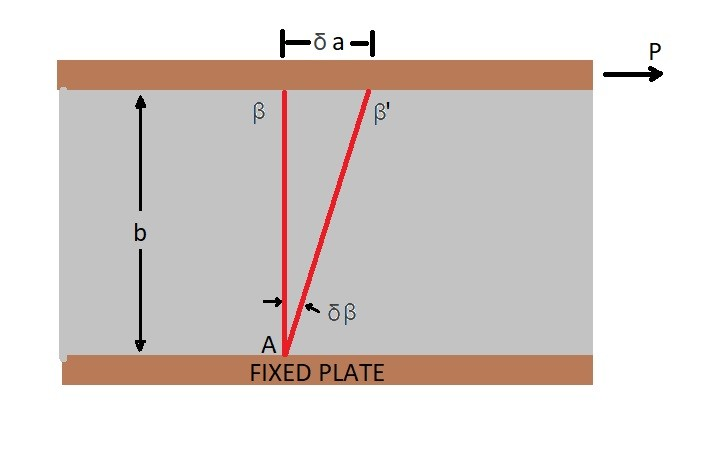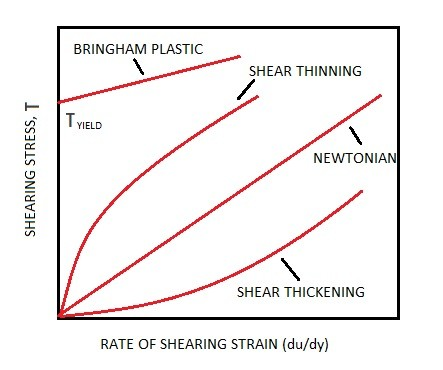# Viscosity

In previous articles I discussed density, which is how heavy a fluid is per unit volume.  However, this is only one aspect that is used to describe a fluid.  As we know fluids flow, and even if they have simular densities, they could flow completely differently.  An example of this is oil and water.  Both oil and water densities are fairly close, with oil having a lighter density. However, when you are observing how the two flow, they are completely different. This is due to viscosity.

### What is Viscosity?

So what is viscosity?  Viscosity if the a property a fluid that describes the stickiness of the fluid.  Let’s take a look at the following example.  You have two infinitely wide plates held at a certain distance that are parallel to each other.  The bottom plate is fixed while top plate is allowed to move.  Now let say you take a piece of metal and glue one end to each plate and than apply a force to the top plate.  After applying this load you would expect the piece of metal to flex in the direction of the load creating shear stress is material as well as deflection $δβ$.  The load can be related to the shear stress using the equation below.Eq 1 $P=τA$

$τ$= shear stress

$A$ = Surface area that contacts fluid

For the above example $δβ$ represents the shearing strain.  The shearing strain is proportional to the shear stress in the material.

Now for the above example let’s replace the solid with a fluid.  Unlike the previous example, when the the force P is applied to the top plate, the top plate will move at a constant velocity.  Notice that I said constant velocity.  This is because as the plate moves a resistance will build up due to the fluid viscosity that will eventually be equal to the force P causing a constant velocity.  As the top plate moves a shear force will develop with in the fluid itself.  The shear forces will cause the fluid to move at a certain velocity, where its velocity will be equal to the top plates velocity at the point where the fluid makes contact with the top plate.  However, it will linearly decrease to zero as the fluid reaches the fixed bottom plate.

You can always expect the fluid velocity to be zero where it makes contact with a fixed surface due to no-slip conditions.  The no-slip condition means that the fluid will stick to solid boundaries, and all  liquids and gases will satisfy this condition.The resulting velocity profile can be related to the shear stress on the fluid by using the following equation.

Eq 2 $τ=μ\frac{du}{dy}$

$μ$ = dynamic viscosity

### Newtonian vs non-Newtonian Fluids

A fluid can either be a Newtonian fluid or a non-Newtonian fluid.  A fluid that is Newtonian will have a linear relation between its shear stress and its shearing strain. While a non-Newtonian fluid will not. Most common liquids and gases will be Newtonian.

The apparent viscosity $μ_{ap}$ is used to determine if the fluid is Newtonian or non-Newtonian.  A Newtonian fluids apparent viscosity will be the same as the dynamics viscosity and will be independent of shear rate.  For non-Newtonian fluids the apparent viscosity will determine if there is shear thickening or shear thinning.

##### Shear Thinning

If there is shear thinning the apparent viscosity will decrease as the shear rate increases causing to the fluid to become less viscous as the shear stress increases. An example of a material that has shear thinning is paint.  At rest a paint’s viscosity is high enough that it will stick to the brush, however it will flow smoothly on the wall when the brush is brushed against it.

##### Shear Thickening

On the other hand if there is shear thickening the apparent viscosity will increase as the shear increases which in turn will cause the fluid to become more viscous as the shear stress increases.  An example of this type fluid would be quicksand.  The faster you try to remove an object from quicksand the harder it is to remove.

##### Bringham Plastic

A matter that has a Bringham plastic behavior is neither a solid or a fluid.  These types of materials will have a certain yield stress that will allow the fluid to act like a solid.  Once these type of material reach their yield stress they will flow like a fluid. An example this wood be tooth paste.  Tooth paste will hold it shape until a certain shear stress which will cause it to flow.